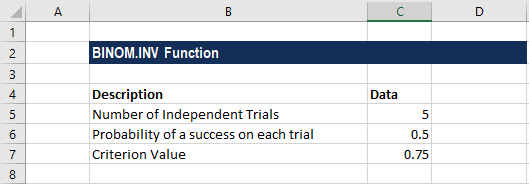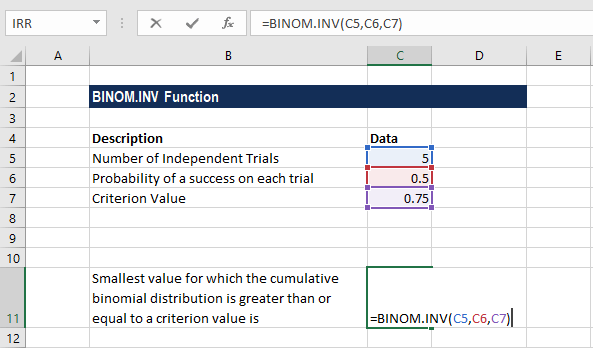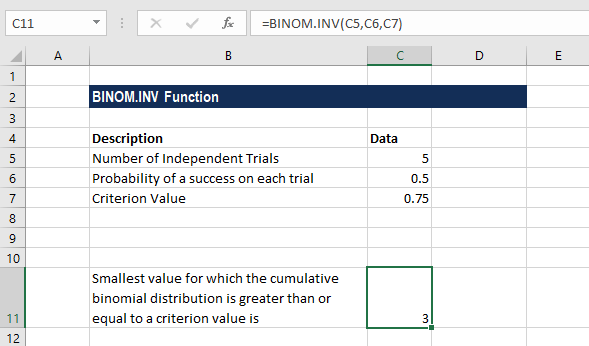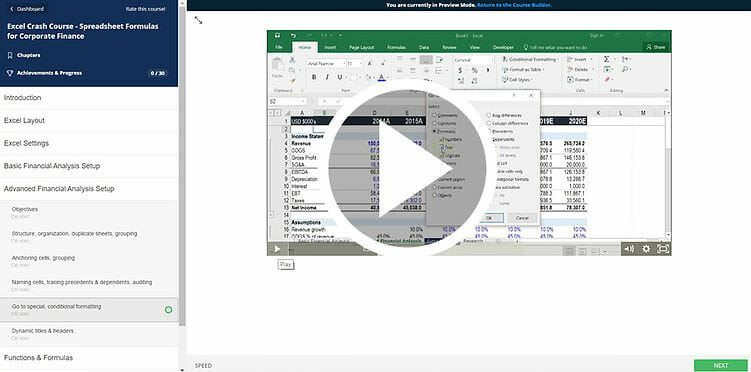# BINOM.INV Function

Calculates the inverse of the Cumulative Binomial Distribution

## Inverse Binomial Distribution in Excel

The BINOM.INV function is categorized under Excel Statistical functions. It will calculate the inverse Binomial Distribution in Excel. That is, for a given number of independent trials, the function will return the smallest value of x (the number of successes) for a specified Cumulative Binomial Distribution probability. For example, we can use it to calculate the minimum number of tosses of a coin required to produce a 50% chance of getting at least 10 heads.

As a financial analyst, BINOM.INV is useful in analyzing securities to invest in. We can use the function to understand how an overall portfolio will be affected by the addition or removal of stocks.

The BINOM.INV function was introduced in MS Excel 2010 and hence is unavailable in earlier versions.

### Formula

=BINOM.INV(trials,probability_s,alpha)

The BINOM.INV function uses the following arguments:

1. Trials (required argument) – This is the number of Bernoulli trials. That is, it is the number of independent trials that are to be done. Excel will truncate the value to an integer if we provide it in decimal form.
2. Probability_s (required argument) – This is the probability of success in a single trial.
3. Alpha (required argument) – This is the probability of Cumulative Binomial distribution. It should be between 0 and 1.

To learn more, launch our free Excel crash course now!

### How to use Inverse Binomial Distribution in Excel

To understand the uses of the BINOM.INV function, let’s consider a few examples:

#### Example

Suppose we are given the following data:Based on the above information, we will calculate the smallest value for which the cumulative binomial distribution is greater than or equal to a criterion value. The formula to use is:We get the result below:So, the minimum number of trials is 3, for which the binomial cumulative distribution is 75%.

### Things to remember about Inverse Binomial Distribution in Excel

1. The BINOM.INV function is an updated version of the CRITBINOM function, which is available in older versions of Excel.
2. #VALUE! error – Occurs when any of the arguments provided is non-numeric.
3. #NUM! error – Occurs when:
• The given trial argument is less than zero.
• The probability_s argument provided is less than zero or greater than 1.
• The given alpha argument is less than zero or greater than 1.

### Free Excel Course

If you want to learn more about functions and become an expert on them, check out our Free Excel Crash Course! Go through our step by step instructions and demonstrations on how to become an Excel power user.### Additional resources

Thanks for reading CFI’s guide to the Excel Inverse Binomial Distribution function. By taking the time to learn and master these functions, you’ll significantly speed up your financial analysis. To learn more, check out these additional CFI resources:

• Excel Functions for Finance
• Advanced Excel Formulas Course
• Advanced Excel Formulas You Must Know
• Excel Shortcuts for PC and Mac

### Free Excel Tutorial

To master the art of Excel, check out CFI’s FREE Excel Crash Course, which teaches you how to become an Excel power user.  Learn the most important formulas, functions, and shortcuts to become confident in your financial analysis.

Launch CFI’s Free Excel Course now to take your career to the next level and move up the ladder!